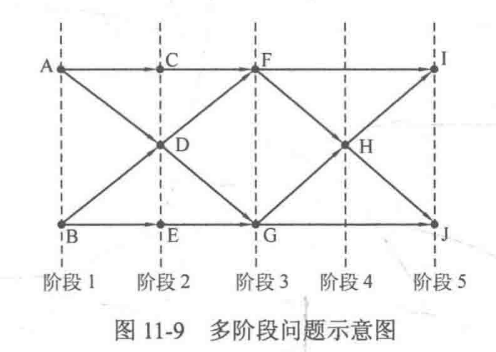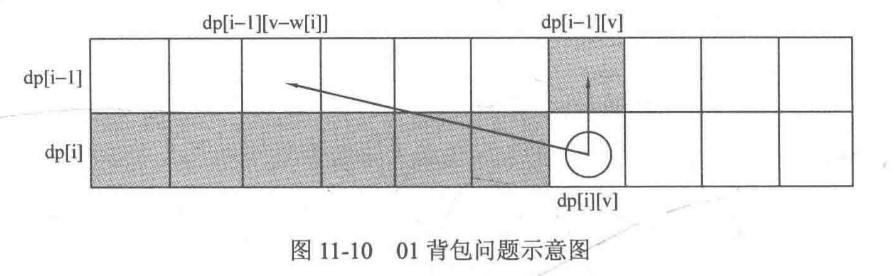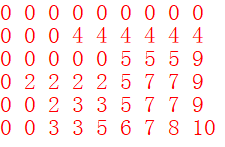# 1. 01背包

01背包问题是经典的 多阶段动态规划 问题。01背包也是如此，前i个物品装入背包的最大价值是由前i-1个物品装入背包的最大价值决定的。

## 问题描述

n件物品，每件物品的体积为w[i]，价值为v[i]，每件物品的体积都不相同，现有一个容量为W的背包，问怎么装才能使得背包内的价值最大？

## 思路

dp[i][j]表示前i件物品装入容量为j的背包中所能获得的最大价值。

1. 如果w[i]>j，即背包放不下第i件物品，显然有dp[i][j] = dp[i-1][j]
2. 如果w[i]<=j，则此时有两种选择，放还是不放。
• 放的话，则dp[i][j] = dp[i-1][j-w[i]] +v[i]
• 不放的话，则dp[i][j] = dp[i-1][j]
• dp[i][j] = max(dp[i-1][j-w[i]] +v[i], dp[i-1][j])

## 代码

### 滚动数组优化## 例子

dp数组为：# 2. 完全背包

## 思路

1. 如果w[i]>j，即背包放不下第i件物品，显然有dp[i][j] = dp[i-1][j]
2. 如果w[i]<=j，则此时有两种选择，放还是不放。
• 不放的话，则dp[i][j] = dp[i-1][j]，这跟01背包是一样的；
• 放的话，就不一样了，因为01背包每种物品只有一件，所以必须转移到dp[i-1][j-w[i]]这个状态，但是完全背包的话每种物品有无穷件，所以放了第i件物品后还可以放第i件物品，故转移到dp[i][j-w[i]]这个状态，所以dp[i][j] = dp[i][j-w[i]]+v[i]
• dp[i][j] = max(dp[i][j-w[i]] +v[i], dp[i-1][j])